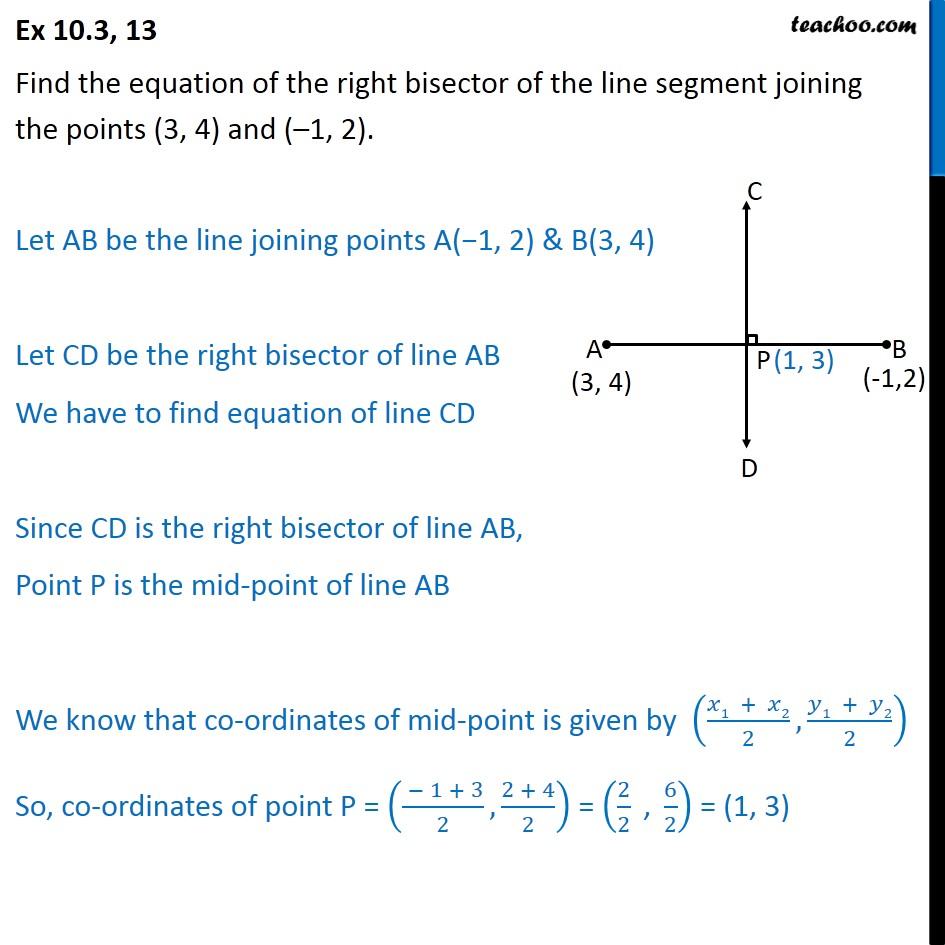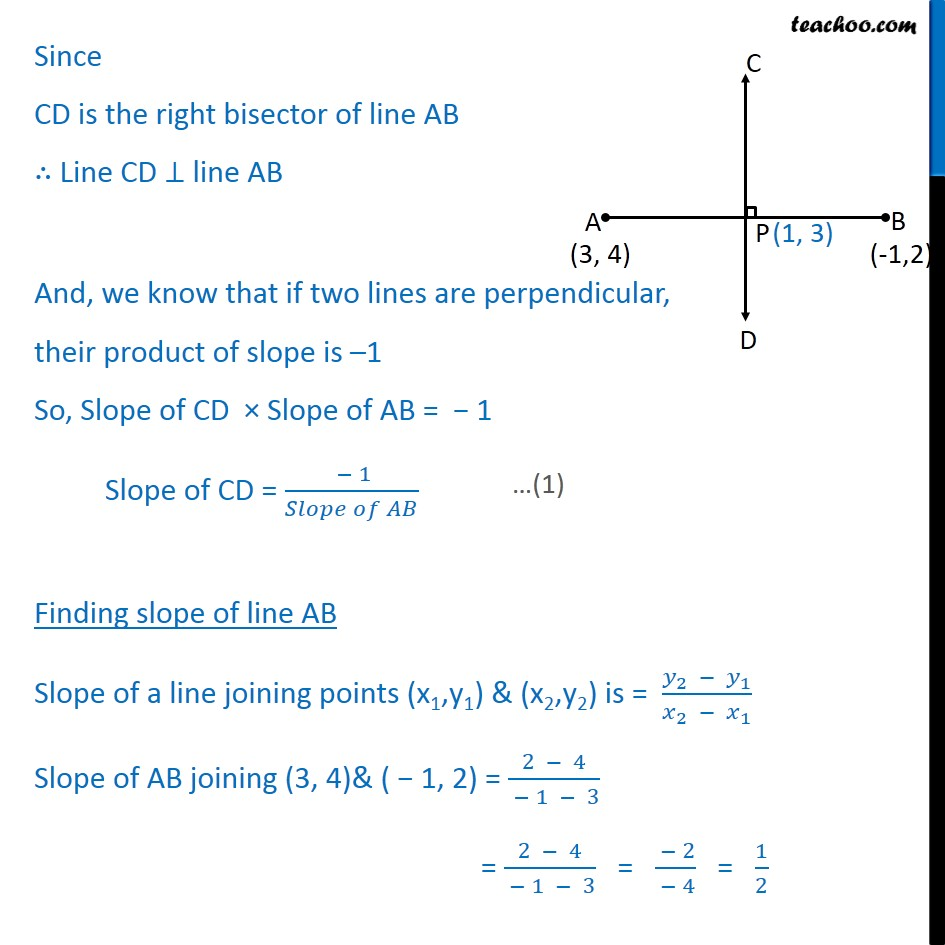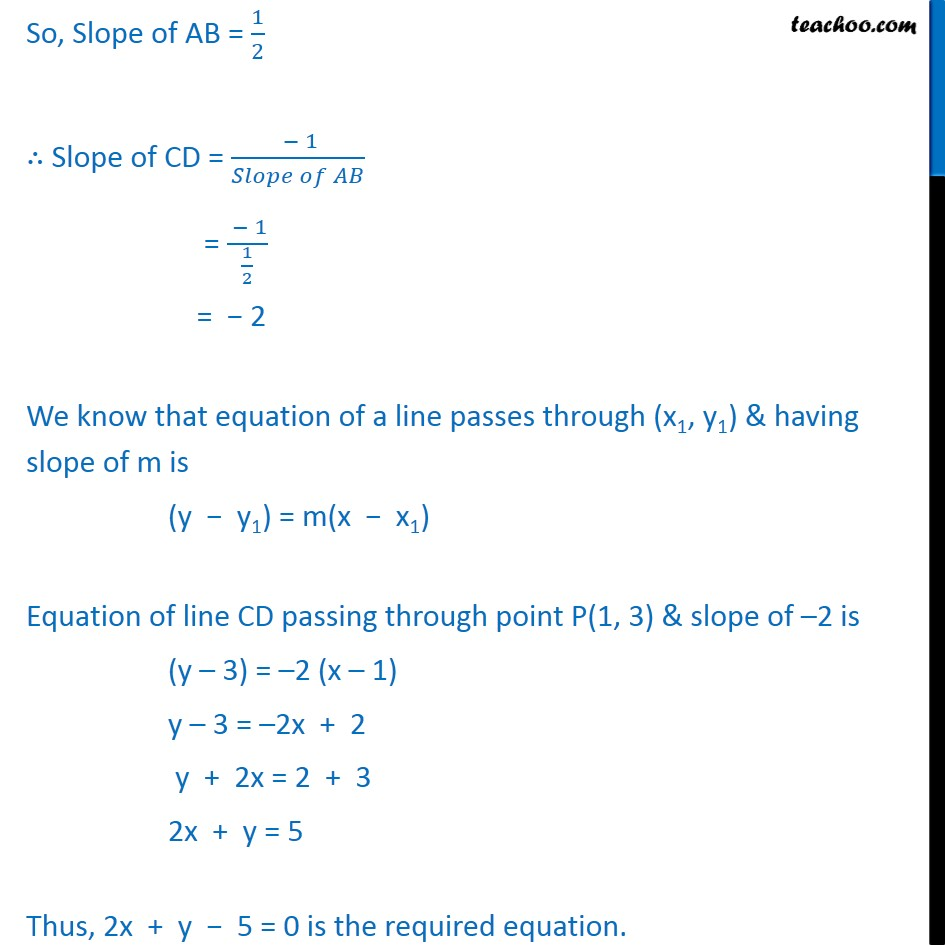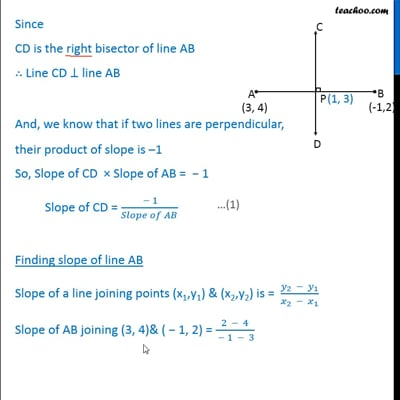Ex 10.3

Chapter 10 Class 11 Straight Lines (Term 1)
Serial order wiseThis video is only available for Teachoo black users

### Transcript

Ex 10.3, 13 Find the equation of the right bisector of the line segment joining the points (3, 4) and ( 1, 2). Let AB be the line joining points A( 1, 2) & B(3, 4) Let CD be the right bisector of line AB We have to find equation of line CD Since CD is the right bisector of line AB, Point P is the mid-point of line AB We know that co-ordinates of mid-point is given by (( 1 + 2)/2, ( 1 + 2)/2) So, co-ordinates of point P = (( 1 + 3)/2, (2 + 4)/2) = (2/2 " , " 6/2) = (1, 3) Since CD is the right bisector of line AB Line CD line AB And, we know that if two lines are perpendicular, their product of slope is 1 So, Slope of CD Slope of AB = 1 Slope of CD = ( 1)/( ) Finding slope of line AB Slope of a line joining points (x1,y1) & (x2,y2) is = ( _2 _1)/( _2 _1 ) Slope of AB joining (3, 4)& ( 1, 2) = (2 4)/( 1 3) = (2 4)/( 1 3) = ( 2)/( 4) = 1/2 So, Slope of AB = 1/2 Slope of CD = ( 1)/( ) = ( 1)/(1/2) = 2 We know that equation of a line passes through (x1, y1) & having slope of m is (y y1) = m(x x1) Equation of line CD passing through point P(1, 3) & slope of 2 is (y 3) = 2 (x 1) y 3 = 2x + 2 y + 2x = 2 + 3 2x + y = 5 Thus, 2x + y 5 = 0 is the required equation.